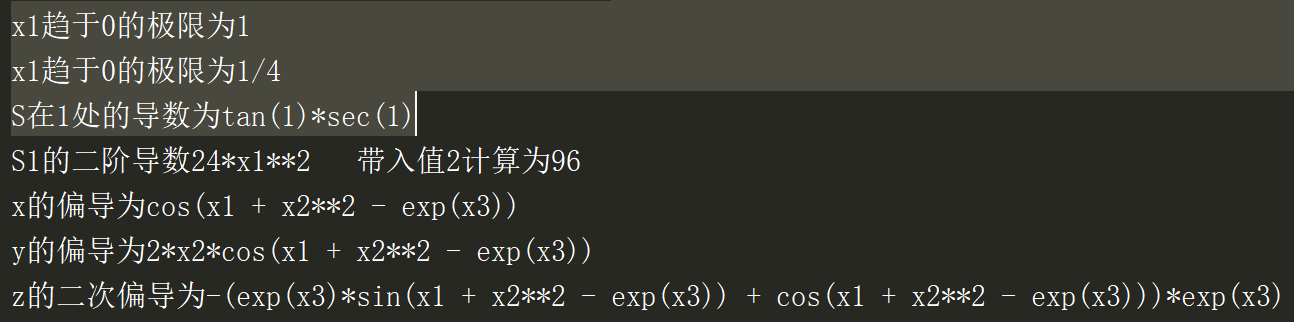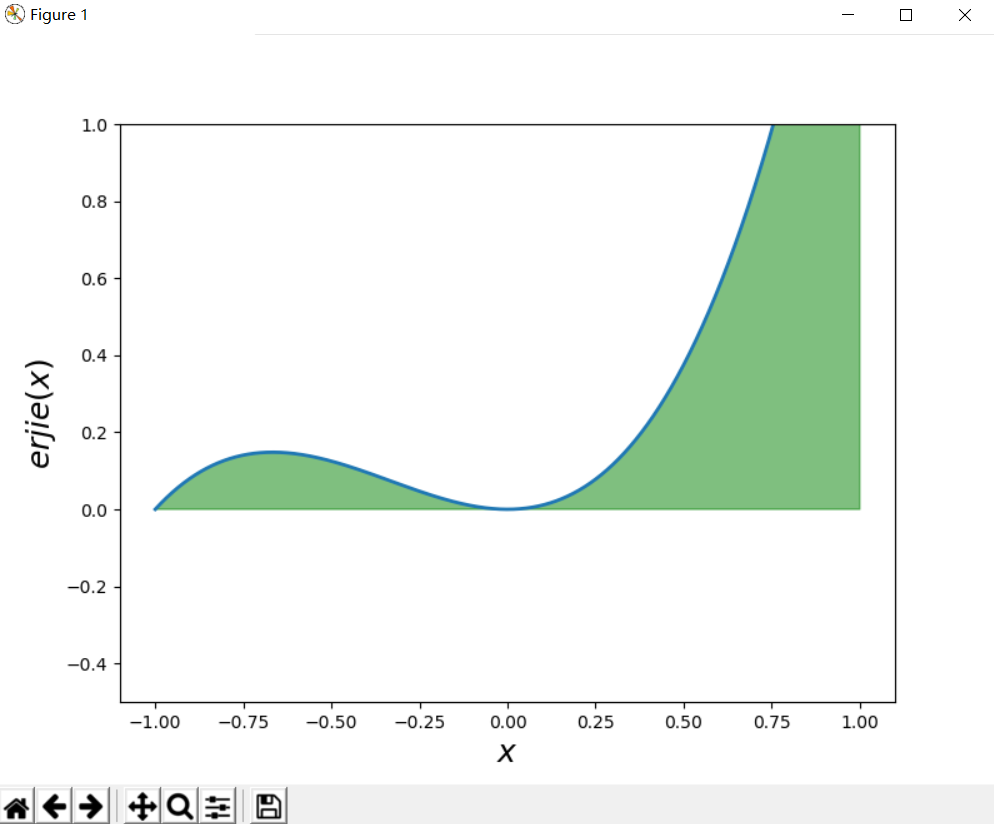• diff(f,x)diff(f, x)diff(f,x)求导数可引入求微分方程 sympy 求微分方程.(点击可跳转) 1.一阶导数 基本格式 print(diff(f, x)) # f为所求导函数，x为对x进行求导 例：求该函数的导数 f(x)=cos(x)f(x)=cos(x)f(x)=...
• 在matlab中求解的代码：>> syms w;F(w)=8*exp(-j*5*w)/(16+w^2);diff(F(w),w,2)ans =- (200*exp(-w*5*i))/(w^2 + 16) - (16*exp(-w*5*i))/(w^2 + 16)^2 + (64*w^2*exp(-w*5*i))/(w^2 + 16)^3 + (w*exp(-w*5*i)...
在matlab中求解的代码：>> syms w;F(w)=8*exp(-j*5*w)/(16+w^2);diff(F(w),w,2)ans =- (200*exp(-w*5*i))/(w^2 + 16) - (16*exp(-w*5*i))/(w^2 + 16)^2 + (64*w^2*exp(-w*5*i))/(w^2 + 16)^3 + (w*exp(-w*5*i)*160*i)/(w^2 + 16)^2>> pretty(ans)264 w  exp(-5 w i)   16 exp(-5 w i)   200 exp(-5 w i)   160 w exp(-5 w i) i----------------- - -------------- - --------------- + -------------------2      3           2      2          2                  2      2(w  + 16)          (w  + 16)          w  + 16           (w  + 16)pretty(ans)为对结果进行美化，写成我们常用的形式。
展开全文• sympy简介:sympy是一个Python的科学计算库，一套强大的符号计算体系完成诸如多项式值、极限、解方程、积分、微分方程、级数展开、矩阵运算等等计算问题。 1、极限、求导、偏导以及带值求导 在这里插入...
sympy简介:sympy是一个Python的科学计算库，用一套强大的符号计算体系完成诸如多项式求值、求极限、解方程、求积分、微分方程、级数展开、矩阵运算等等计算问题。
1、求极限、求导、求偏导以及带值求导
在这里插入代码import sympy
#求极限
#设置符号变量Symbol只能创建一个变量 symbols 可一次定义多个变量
x1,x2,x3,x4=sympy.symbols('x1,x2,x3,x4')
#创建函数建立方程式
def F(t):
return sympy.sin(t)/t
def N(t):
return (x1**3+3*x1**2+1)/(4*x1**3+2*+3)
#调用limit求极限
limF=sympy.limit(F(x1),x1,0)
limN=sympy.limit(N(x1),x1,sympy.oo)
print("x1趋于0的极限为{}".format(limF))
print("x1趋于0的极限为{}".format(limN))
#求导
#创建求导函数
def S(t):
return sympy.sec(t) #正割
def S1(x):
return 2*x**4+2
#调用diff函数求导
s=sympy.diff(S(x1),x1).subs(x1,1) #subs 带值求导
print('S在1处的导数为{}'.format(s))
#求多阶导数 2阶
s1=sympy.diff(S1(x1),x1,2)
#带值计算
print("S1的二阶导数{}   带入值2计算为{}".format(s1,s1.subs(x1,2)))
#建立求偏导函数
def PD(x,y,z):
return sympy.sin(x+pow(y,2)-sympy.exp(z))
#对x求偏导
x=sympy.diff(PD(x1,x2,x3),x1)
# print(x.subs(x1,2))
#对y求偏导
y=sympy.diff(PD(x1,x2,x3),x2)
#对z求偏导
z=sympy.diff(PD(x1,x2,x3),x3,2)
print("x的偏导为{}\ny的偏导为{}\nz的二次偏导为{}".format(x,y,z))
片

结果如下2、求极值 并画出函数图像
import sympy
import math
import matplotlib.pyplot as plt
import numpy as np

#设置符号变量Symbol只能创建一个变量 symbols 可一次定义多个变量
x1,x2,x3,x4=sympy.symbols('x1,x2,x3,x4')

#用二阶导求极限
def erjie(x):
return  x**3+x**2
x2=sympy.diff(erjie(x1),x1) #求一阶导
bb=sympy.solve(x2,x1)
x3=sympy.diff(erjie(x1),x1,2)  #求二阶导
ll = []
LL=[]
for i in bb:
if x3.subs(x1,i)>0:
LL.append(erjie(x1).subs(x1,i))  #二阶导大于零取极小值 小于零取极大值
if x3.subs(x1,i)<0:
ll.append(erjie(x1).subs(x1,i))   #可以直接erjie(i)
print("极小值",LL)
print("极大值",ll)
#绘制图像
fig,ax=plt.subplots(figsize=(8,6))
x=np.linspace(-1,1,10000)
ax.plot(x,erjie(x),lw=2)
ax.fill_between(x,erjie(x),color='green',alpha=0.5)
ax.set_xlabel("$x$",fontsize=18)
ax.set_ylabel("$erjie(x)$",fontsize=18)
ax.set_ylim(-0.5,1)
# plt.show()
plt.draw()
plt.pause(3)# 间隔的秒数：3s
plt.close(fig)

运行结果展开全文• 在要获得导数的精确近似值，需要使h相当小。但是，如果将其设置得太小，那么实际上会由于Python浮点的精度有限而失去准确性。使用decimal模块可以获得更高的精度。但是，该模块只支持简单的算术运算符和平方根函数。...
“某人”已经回答了您的问题，但您(以及未来的读者)可能会发现这些补充信息很有用。在要获得导数的精确近似值，需要使h相当小。但是，如果将其设置得太小，那么实际上会由于Python浮点的精度有限而失去准确性。使用decimal模块可以获得更高的精度。但是，该模块只支持简单的算术运算符和平方根函数。如果您需要三角函数或指数函数之类的函数，那么您可以使用第三方软件包任意精度的数学软件包，例如优秀的^{}，尽管如果您确实使用mpmath，那么您可能会使用它的numerical derivative functions。在FWIW，您可以通过使x作为区间的中间，使导数近似更精确(对于给定的h)。下面是一个简短的演示：def dfa(f, x, h):return (f(x + h) - f(x)) / hdef dfb(f, x, h):hh = 0.5 * hreturn (f(x + hh) - f(x - hh)) / h# The functiondef func(x): return x**3 + x*x + x + 1# Its exact derivativedef dfunc(x): return 3*x*x + 2*x + 1h = 0.001for i in range(10):x = 1 + 0.1 * iprint(x, dfunc(x), dfb(func, x, h), dfa(func, x, h))输出^{pr2}\$这是指数函数的结果。在from math import expdef dfa(f, x, h):return (f(x + h) - f(x)) / hdef dfb(f, x, h):hh = 0.5 * hreturn (f(x + hh) - f(x - hh)) / hfunc = dfunc = exph = 0.001for i in range(10):x = 1 + 0.1 * iprint(x, dfunc(x), dfb(func, x, h), dfa(func, x, h))输出1.0 2.718281828459045 2.718281941720413 2.71964142253322551.1 3.0041660239464334 3.0041661491195804 3.0056686077775121.2 3.3201169227365472 3.320117061074157 3.32177753468876351.3 3.6692966676192444 3.669296820505874 3.67113192765478051.4 4.0551999668446745 4.0552001358102885 4.0572282428632531.5 4.4816890703380645 4.481689257074706 4.4839306620083621.6 4.953032424395115 4.953032630771403 4.9555097663187551.7000000000000002 5.473947391727201 5.473947619807795 5.4766852779755131.8 6.0496474644129465 6.049647716480422 6.05267329667125151.9 6.6858944422792685 6.685894720857455 6.689238504094419注意dfb并不是万无一失的：如果您试图寻找一个靠近极点或间断点的x值的导数，那么改变区间以包含极点或间断可能会产生错误的结果。当然，dfa也会遇到同样的问题，例如如果f(x) = 1/x和{}是负数，而{}是正数。在
展开全文• 当你认为一个常数(关于某事物)的(偏)导数是0时，这是显而易见的。在Python中，可以使用符号数学模块(如SymPy或SymEngine)计算函数的jacobian。以下是维基百科的一个简单示例：使用SymEngine模块：Python 2.7.11 (v...
Jacobian仅为向量值函数定义。不能使用填充有常数的数组来计算雅可比；必须知道基本函数及其偏导数，或它们的数值近似值。当你认为一个常数(关于某事物)的(偏)导数是0时，这是显而易见的。在Python中，可以使用符号数学模块(如SymPy或SymEngine)计算函数的jacobian。以下是维基百科的一个简单示例：使用SymEngine模块：Python 2.7.11 (v2.7.11:6d1b6a68f775, Dec  5 2015, 20:40:30) [MSC v.1500 64 bit (AMD64)] on win32Type "help", "copyright", "credits" or "license" for more information.>>>>>> import symengine>>>>>>>>> vars = symengine.symbols('x y') # Define x and y variables>>> f = symengine.sympify(['y*x**2', '5*x + sin(y)']) # Define function>>> J = symengine.zeros(len(f),len(vars)) # Initialise Jacobian matrix>>>>>> # Fill Jacobian matrix with entries... for i, fi in enumerate(f):...     for j, s in enumerate(vars):...         J[i,j] = symengine.diff(fi, s)...>>> print J[2*x*y, x**2][5, cos(y)]>>>>>> print symengine.Matrix.det(J)2*x*y*cos(y) - 5*x**2
展开全文• 在前面的笔记里孤光一点萤：数值常微分方程-欧拉法与龙格-库塔法​zhuanlan.zhihu.com整理了常微分方程的一些数值解法。...同样地，在计算机里无法取极限求导数，只能通过离散化之后，有限差商...
• 目录导数数值微分偏导数梯度（gradient） 导数 一个函数在某一点的导数描述了这个...python示例：分别数值微分和解析求导计算f=x2在x=2处的导数： #定义函数f def f(x): return x*x # 定义数值微分计算方法 def n
• 梯度下降也可以用导数知识轻松解释。实时证明沉寂了一年多的我去考研，不碰代码。对我来说完全是个正确的决定。线性回归线性：y=a*x 一次方的变化回归：回归到平均值简单线性回归算法==公式一元一次方程组一...
• 文章目录sympy库极限导数 sympy库 极限 import sympy #求极限 x=sympy.Symbol('x') #定义变量 y=x**2 #定义函数 ...#求导数 x=sympy.Symbol('x') #定义变量 y=x**2 #定义函数 data=sympy.diff(y,x,1...
• 用Python玩转数据_答案答案：更多相关问题由参数方程所确定的函数y=y(x)的二阶导数已知数列的通项公式,则取最小值时=,此时=．(本小题满分10分)已知是等差数列，其中](1)的通项;(2)数列从哪一项开始小于0;(3)值...
• 我们在衡量某个函数敏感度与稳定性时，常使用的一种方法是求导。即观察导数 的大小。然而对于一个矩阵而言，所谓的导数似乎就不是那么显著，在此我们就介绍一个常用的...那么我们就可以解x的相对误差与b的相对误...
• 不过有人居然用Python轻松解决了,相信大家一定很好奇吧!问题背景高等数学应用非常广，基本上涉及到函数的地方都要用到微积分，还有在几何方面也是如此，计算机的应用让我们能简单快速处理各种高等数学中的计算，比如...
• 接下来用Python实现最土的梯度下降算法, #首先引入numpy模块和图形界面模块 import matplotlib.pyplot as plt import numpy as np #定义三个函数 一个数目标函数 一个是对目标函数x1一次导数和x2一次导数 def ...
• 优化算法经常要用到导数、梯度、Hesse矩阵等，因此编写了一个类用于实现这些功能 建立一个Function类，构造函数的参数是一个函数 其中part的功能是偏导，var_index表示是第几个变量，val表示这些变量的值 ...
• 中心差分法详见：数值微分|中心差分法(Central Finite Difference Approximations)区间端点的导数时,不能中心差分法。考虑在个离散点给出函数的情况,由于中心差分在的两侧使用函数的值，因此我们将无法计算导数...
• 上一篇文章【机器学习】如何用Python书写计算任一函数任一点的导数？，只介绍了单一变量的函数求导Python实现，而实际的问题中遇到大多都是多变量情况，但是知道偏导的概念后，再结合Python的特性就很容易解决如何...
• 在所有有限差分表达式中，系数之和为...为了解决这个矛盾，我们可以采取以下措施：1 使用双精度浮点数运算2 采用精确度至少为的有限差分公式例如，中心差分法计算 在 处的二阶导数。取不同的 值以及精度为 和 ，手...
• 摘要： 实际问题中主要涉及的还是多变量的函数，单一变量导数的计算其实是多变量导数求偏导的一...)前言上一篇文章如何用Python书写计算任一函数任一点的导数？，只介绍了单一变量的函数求导Python实现，而实际的问...
• 梯度下降是机器学习里的一个重要的方法，... 在这样的一元线性函数中，m，b的值要通过求导数的方法获的，求导数也就是求X，Y在连续变化下的切点的变化率。 如果是多元线性函数，叫求偏导(假设其他元的变量不变)下...
• 考研数学中会涉及到很多微积分的题目。微积分是高等数学最重要的概念，对于这么重要的数学工具，python肯定不会错过。我们不难找到，在sympy这个python数学计算模块中，...一、高阶导数 from sympy import Deriv......

# 用python求导数python 订阅